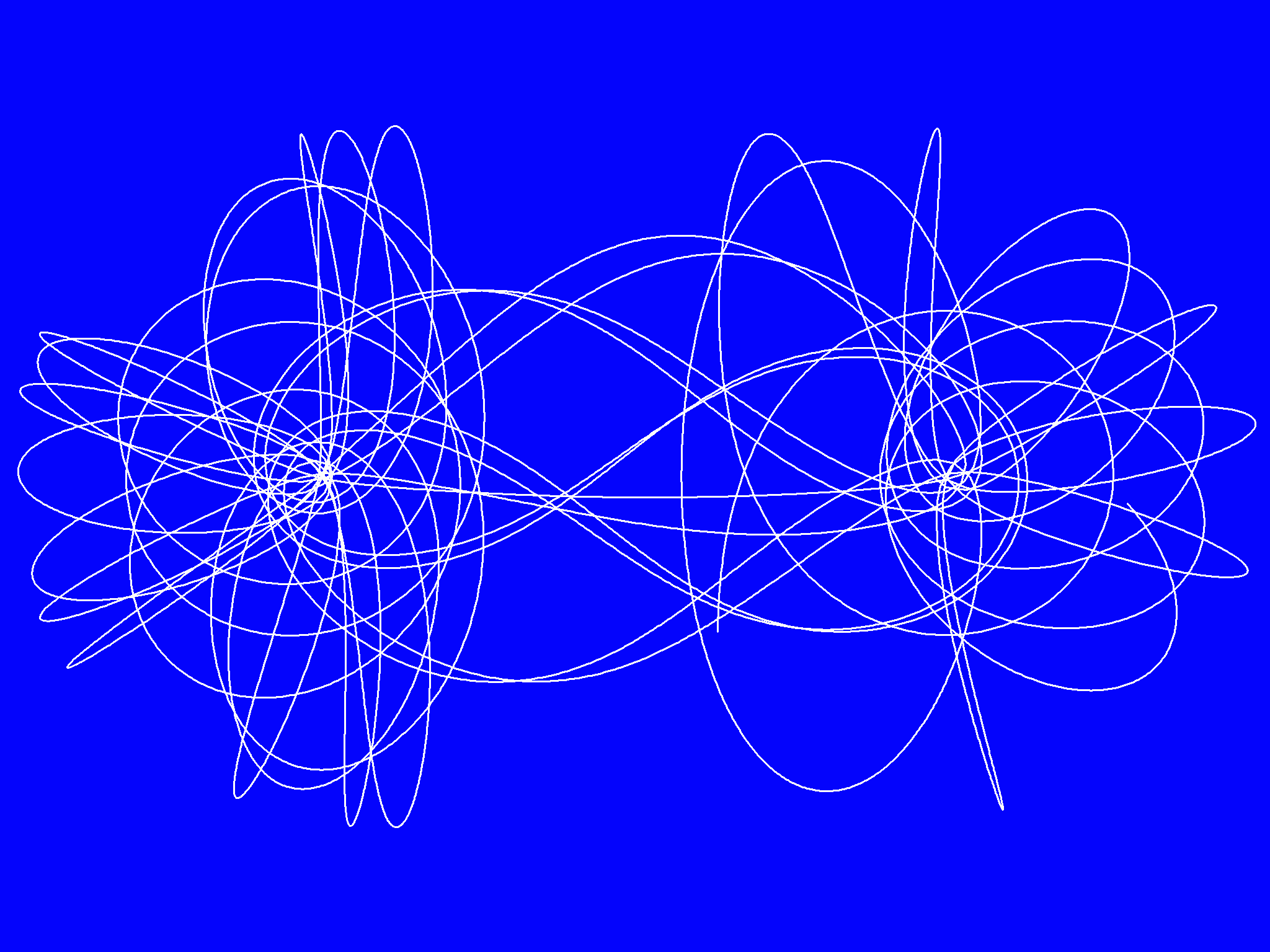# Restricted Three-Body Problem

J. C. Sprott
Department of Physics, University of Wisconsin, Madison, WI 53706, USA
September 13, 2007

The three-body problem (a planet orbiting a pair of stars, for example) is one of the oldest problems in all of mathematical physics. It has never been solved in the usual sense of solving a mathematical problem, namely finding an equation to predict where the planet will be at some future time for a given set of initial conditions. The reason is that the motion is chaotic and exhibits sensitive dependence on initial conditions. The problem can, of course, be solved numerically, and the equations are not difficult. Shown here is the solution for a highly restricted version of the 3-body problem in which the two point-like stars are fixed and equally massive relative to the planet, which orbits the stars in a plane. Since the orbit typically approaches arbitrarily close to the stars, to make the problem numerically tractable, the stars are given a small diameters (5% of their separation), inside of which the gravitational force varies linearly with the distance from their centers rather than as the inverse square. The calculations were done in PowerBASIC whose source and object code are available for inspection. A high resolution plot of the resulting orbit is shown below: